# 【Python基础】第九课：使用Pandas和Numpy处理数据

## Pandas，NumPy，zip函数，列表解析，NumPy数组，DataFrame，Series

Posted by x-jeff on December 29, 2019

# 1.简介

## 1.1.Pandas

Pandas是一个强大的分析结构化数据的工具集；它的使用基础是Numpy（提供高性能的矩阵运算）；用于数据挖掘和数据分析，同时也提供数据清洗功能。

## 1.2.Numpy

NumPy是使用Python进行科学计算的基础软件包。除其他外，它包括：

• 功能强大的N维数组对象。
• 精密广播功能函数。
• 集成 C/C+和Fortran 代码的工具。
• 强大的线性代数、傅立叶变换和随机数功能。

• 英文官网：numpy
• 中文官网：NumPy中文

# 2.NumPy基本用法

1
2
a=[1,2,3]
b=[2,3,4]


1
2
for k,v in zip(a,b):
print(k*v)


1
2
3
2
6
12


1
[k*v for k,v in zip(a,b)] #输出为[2,6,12]


1
2
3
4
5
import numpy as np
a=np.array([1,2,3])
b=np.array([2,3,4])
print(a+b) #输出为[3 5 7]
print(a*b) #输出为[2 6 12]


## 2.1.关于zip的用法

1
2
3
4
5
6
7
8
9
10
a=[1,2,3]
b=[4,5,6]
c=[4,5,6,7,8]
zipped1=zip(a,b)
print(list(zipped1)) #zipped1为[(1, 4), (2, 5), (3, 6)]
zipped2=zip(a,c)
print(list(zipped2)) #zipped2为[(1, 4), (2, 5), (3, 6)]
d1,d2=zip(*zip(a,b))
print(list(d1)) #d1为[1, 2, 3]
print(list(d2)) #d2为[4, 5, 6]


⚠️需要注意四点：

1. Python2中zip()返回的是一个列表，可以直接print(zipped1)，但是在Python3中，为了减少内存，zip()返回的是一个对象，如需展示列表，需手动list()转换，即print(list(zipped1))
2. 如果各个迭代器的元素个数不一致，则返回列表长度与最短的对象相同。
3. 利用*号操作符，可以将元组解压为列表。
4. zip对象在使用一次后就会失效清空，例如：
1
2
3
4
5
a=[1,2,3]
b=[4,5,6]
c=zip(a,b)
d1,d2=zip(*c)#正常无报错
d1,d2=zip(*c)#c已经使用过一次了,本语句会报错


## 2.2.列表解析

1
listA=[a for a in range(1,5)]


⚠️listA=[1,2,3,4]而不是listA=[1,2,3,4,5]

# 3.Pandas基本用法

## 3.1.DataFrame

DataFrame是Pandas中的一个表格型的数据结构，包含有一组有序的列，每列可以是不同的值类型（数值、字符串、布尔型等），DataFrame即有行索引也有列索引，可以被看做是由Series组成的字典。

1
2
import pandas as pd
df=pd.DataFrame([['Frank','M',29],['Mary','F',23],['Tom','M',35],['Ted','M',33],['Jean','F',21],['Lisa','F',20]])


df的样式为：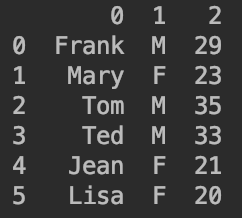1
df.columns=['name','gender','age']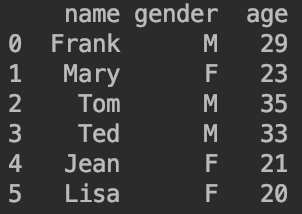### 3.1.1.快速查看DataFrame的信息

👉查看df的有关信息df.info()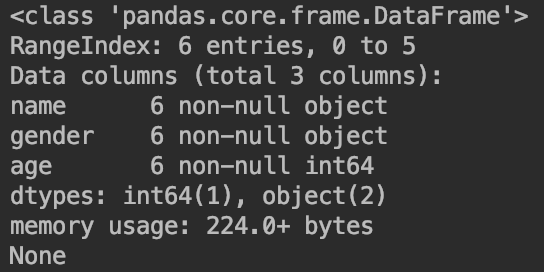👉查看df的前几行和后几行（默认查看5行）：

1
2
3
4
df.tail() #查看后5行
df.tail(3) #查看后3行


👉查看有关df的叙述性统计（针对连续型数据）df.describe()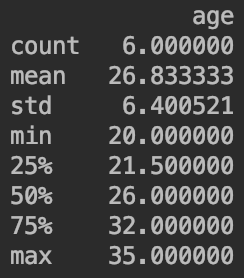df["age"].describe().mean()输出的是统计信息（数据条数、均值、标准差等）的均值，而不是age属性的均值。

## 3.2.Series

1
type=pd.Series([21,18,35])


type输出为：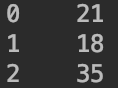1
type=pd.Series([21,18,35],index=['A','B','C'])


type输出为：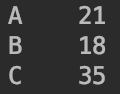1
2
type #按位置访问，输出为18
type['A'] #输出为21


type[0:2]输出为前两行的数据：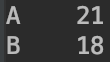❗️多维的Series组合在一起就成为了DataFrame。

# 5.参考资料